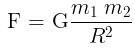Courses

# Test: Universal Law of Gravitation

## 10 Questions MCQ Test Physics Class 11 | Test: Universal Law of Gravitation

Description
This mock test of Test: Universal Law of Gravitation for JEE helps you for every JEE entrance exam. This contains 10 Multiple Choice Questions for JEE Test: Universal Law of Gravitation (mcq) to study with solutions a complete question bank. The solved questions answers in this Test: Universal Law of Gravitation quiz give you a good mix of easy questions and tough questions. JEE students definitely take this Test: Universal Law of Gravitation exercise for a better result in the exam. You can find other Test: Universal Law of Gravitation extra questions, long questions & short questions for JEE on EduRev as well by searching above.
QUESTION: 1

### SI unit of gravitational constant is __________.

Solution:

We know that:Thus we get, [G] = [F.R2 / m2 ] = [F] x L2 x M-2 = [F] M-2 L= N kg-2 m2

QUESTION: 2

### The dimensions of gravitational constant G is:

Solution:

From Newton’s law of gravitation,Since, Force (F) = Mass × Acceleration = M × [LT-2]
∴ The dimensional formula of force = M1 L1 T-2

⇒ Gravitational Constant (G) = F × r2 × [m1m2]-1
Or, G = [M1 L1 T-2] × [L]2 × [M]-2 = [M-1 L3 T-2].

Therefore, the gravitational constant is dimensionally represented as [L][M]-1 [T]-2.

QUESTION: 3

### The mass of the body on moon is 40kg, what is the weight on the earth.

Solution:

Weight = Mass * Accelertion due to gravity = 40 * 9.8 = 392 N

QUESTION: 4

The tides are formed due to:

Solution:

Tides refer to the rise and fall of our oceans' surfaces. It is caused by the attractive forces of the Moon and Sun's gravitational fields as well as the centrifugal force due to the Earth's spin.

QUESTION: 5

Two bodies with same mass “m” separated by a distance “r” exert a gravitational force of F on each other. Suppose the distance between them is doubled and the force becomes F’. The ratio of two forces is

Solution:

We know that the force of gravitation is inversely proportional to square of the distance between the two bodies,

i.e. F∝ r-2

Hence, when the distance between them will be doubled, the force will be reduced by 4 times

So, the ratio will be 4:1

QUESTION: 6

What is the value of G in cgs system of units?

Solution:

The value of G is universally constant = 6.67 × 10-8 dyne.cm2/g2

We know:
1 dyne = 10-5 N
1 cm = 10-2 m
1 g = 10-3 kg

⇒ 6.67 × 10-8 × ( 10-5) .(10-2)2/(10-3)2 Nm2/kg2 = 6.67 × 10-8 { 10-5× 10-4 /10-6} Nm2/kg2
= 6.67 × 10-8 × 10-3 N.m2/kg2= 6.67× 10-11 Nm2/kg2

QUESTION: 7

Gravitation follows:

Solution:
• Gravitational force strictly follows Newton’s Third Law of motion i.e. for every action force there is an equal and opposite reaction force.
• Gravitational forces add up like vectors i.e. they obey the principle of superposition.
QUESTION: 8

G is a universal gravitational constant, its value is:

Solution:

We know that: [g] = [G.m.m / r.r]
Since the unit of G must be same as the unit of g.r.r / m.m  i.e. Nm2 /kg2

Also, we know that G is very very less than 1 as the gravitational force is a very weak force.

QUESTION: 9

In orbit, objects inside a spacecraft feel weightless:

Solution:
• Astronauts float around in space because there is no gravity in space.
• Astronauts are so far from the Earth that gravity is so small. This is why NASA calls it microgravity.
QUESTION: 10

What pulls a ball back to earth?

Solution:

The gravitational force of the earth acts on every object having mass. When a ball is thrown upwards the gravitational force of the earth acts against the velocity of the object and pulls the ball downwards, i.e, towards itself.

Thus, gravity pulls a ball back to earth.